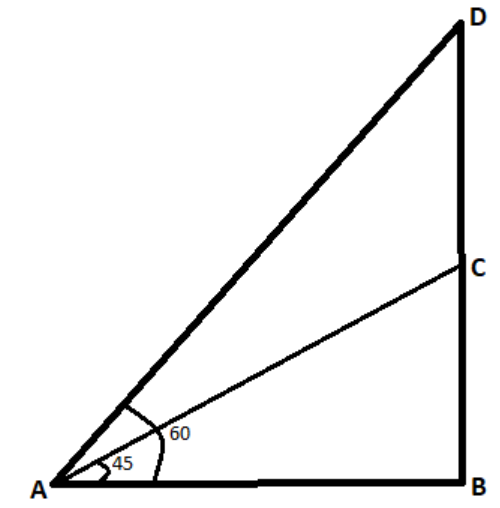Courses
Courses for Kids
Free study material
Free LIVE classes
MoreLIVE
Join Vedantu’s FREE Mastercalss

# A flagstaff stands on the top of a 5m high tower. From a point on the ground, the angle of elevation on the top of the flagstaff is 60° and from the same point, the angle of elevation of the top of the top of the tower is 45°. Find the height of the flagstaff.Verified
265.7k+ views
Hint – In order to solve the given question we draw an appropriate figure using the given data. Then observing the figure, we use the definitions of trigonometric functions to find out the unknown value.

Given data, height of the tower = 5m
Angle of elevation on the top of flagstaff = 60°
Angle of elevation on the top of tower = 45°Let BC be the height of the tower.
Let DC be the height of the flag staff.
Let the point on the ground be A.

In right △ABC,
The right angle is at B.

We know that cotθ =$\dfrac{{{\text{adjacent side}}}}{{{\text{opposite side}}}}$.
From the figure,
AB = BC cot 45°
Given height of the tower is 5m, i.e. BC =5m
AB = 5 x 1 (cot45° = 1)
AB = 5m - (1)

In the right angled triangle △ABD,
The right angle is at B.

We know that cotθ =$\dfrac{{{\text{adjacent side}}}}{{{\text{opposite side}}}}$.
From the figure,
AB = BD cot 60°
AB = (BC+CD) cot 60° (cot 60° = $\dfrac{1}{{\sqrt 3 }}$)
AB = (5+CD) $\dfrac{1}{{\sqrt 3 }}$ ........ (ii)

Equating (i) and (ii), we get
(5 + CD) $\dfrac{1}{{\sqrt 3 }}$ = 5
(5 + CD) = 5$\sqrt 3$
CD = 5$\sqrt 3$-5 = 5(1.732-1) = 5 × 0.732 = 3.66 m ($\sqrt 3$=1.732)
Therefore the height of the flag - staff is 3.66 m.

Note: In order to solve this kind of question, the key is to carefully translate the given sentences into a diagram. The use of trigonometric equations is an important step.
A right angled triangle is a triangle in which one angle is a right angle (that is, a 90-degree angle). The relation between the sides and angles of a right triangle is the basis for trigonometry.
Last updated date: 01st Oct 2023
Total views: 265.7k
Views today: 7.65k